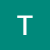# An interesting Coding Problem : Smallest Range II

Hi there!

Firstly the link to the problem I want to discuss is :

I suggest you to give it a try and comeback.

problem statement :

Given an array `A` of integers, for each integer `A[i]` we need to choose either `x = -K` or `x = K`, and…

# 5 cool PyTorch Functions that are beginner-friendly

PyTorch is a Python library with a wide variety of functions and operations, mostly used for deep learning. Data is stored in tensors. A tensor is a vector, number, matrix, or an n-dimensional array. Let’s see a few simple functions which can come in handy for you.

• torch.randn
• torch.cat
• torch.reshape